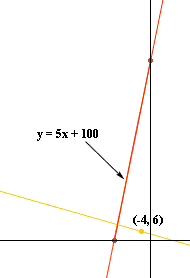Quandaries and Queries My name is Amy My level is college algebra I am a student My problem is: Write the equation of the line that contains the point (-4,6) and is perpendicular to the line y=5x+100. Hi Amy, I would first draw a diagram, even if it is only a mental diagram in my head.The fact you need is that if two lines are perpendicular then the product of their slopes is -1. The slope of the line y = 5x + 100 is 5 and thus the slope of a perpendicular line is -1/5. Can you complete it now? Penny Go to Math Central